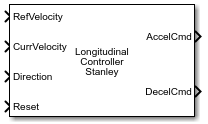# Longitudinal Controller Stanley

Control longitudinal velocity of vehicle by using Stanley method

• Library:
• Automated Driving Toolbox / Vehicle Control

•## Description

The Longitudinal Controller Stanley block computes the acceleration and deceleration commands, in meters per second, that control the velocity of the vehicle. Specify the reference velocity, current velocity, and current driving direction. The controller computes these commands using the Stanley method , which the block implements as a discrete proportional-integral (PI) controller with integral anti-windup. For more details, see Algorithms.

You can also compute the steering angle command of a vehicle using the Stanley method. See the Lateral Controller Stanley block.

## Ports

### Input

expand all

Reference velocity, in meters per second, specified as a real scalar.

Current velocity of the vehicle, in meters per second, specified as a real scalar.

Driving direction of vehicle, specified as `1` for forward motion and `-1` for reverse motion.

Trigger to reset the integral of velocity error, e(k), to zero. A value of `0` holds e(k) steady. A nonzero value resets e(k).

### Output

expand all

Acceleration command, returned as a real scalar in the range [0, MA], where MA is the value of the Maximum longitudinal acceleration (m/s^2) parameter.

Deceleration command, returned as a real scalar in the range [0, MD], where MD is the value of the Maximum longitudinal deceleration (m/s^2) parameter.

## Parameters

expand all

Proportional gain of controller, Kp, specified as a positive real scalar.

Integral gain of controller, Ki, specified as a positive real scalar.

Sample time of controller, in seconds, specified as a positive real scalar.

Maximum longitudinal acceleration, in meters per second squared, specified as a positive real scalar.

The block saturates the output from the AccelCmd to the range [0, MA], where MA is the value of this parameter. Values above MA are set to MA.

Maximum longitudinal deceleration, in meters per second squared, specified as a positive real scalar.

The block saturates the output from the DecelCmd port to the range [0, MD], where MD is the value of this parameter. Values above MD are set to MD.

## Algorithms

The Longitudinal Controller Stanley block implements a discrete proportional-integral (PI) controller with integral anti-windup, as described by the Anti-windup method (Simulink) parameter of the PID Controller block. The block uses this equation:

`$u\left(k\right)=\left({K}_{\text{p}}+{K}_{\text{i}}\frac{{T}_{\text{s}}\text{\hspace{0.17em}}z}{z-1}\right)\text{\hspace{0.17em}}e\left(k\right)$`
• u(k) is the control signal at the kth time step.

• Kp is the proportional gain, as set by the Proportional gain, Kp parameter.

• Ki is the integral gain, as set by the Integral gain, Ki parameter.

• Ts is the sample time of the block in seconds, as set by the Sample time (s) parameter.

• e(k) is the velocity error (CurrVelocityRefVelocity) at the kth time step. For each k, this error is equal to the difference between the current velocity and reference velocity inputs (CurrVelocityRefVelocity).

The control signal, u, determines the value of acceleration command AccelCmd and deceleration command DecelCmd. The block saturates the acceleration and deceleration commands to respective ranges of [0, MA] and [0, MD], where:

• MA is value of the Maximum longitudinal acceleration (m/s^2) parameter.

• MD is the value of the Maximum longitudinal deceleration (m/s^2) parameter.

At each time step, only one of the AccelCmd and DecelCmd port values is positive, and the other port value is `0`. In other words, the vehicle can either accelerate or decelerate in one time step, but it cannot do both at one time.

The direction of motion, as specified in the Direction input port, determines which command is positive at the given time step.

Direction Port ValueControl Signal Value u(k)AccelCmd Port ValueDecelCmd Port ValueDescription
`1` (forward motion)

u(k) > 0

positive real scalar`0`Vehicle speeds up as it travels forward

u(k) < 0

`0`positive real scalarVehicle slows down as it travels forward
`-1` (reverse motion)

u(k) > 0

`0`positive real scalarVehicle slows down as it travels in reverse

u(k) < 0

positive real scalar`0`Vehicle speeds up as it travels in reverse

 Hoffmann, Gabriel M., Claire J. Tomlin, Michael Montemerlo, and Sebastian Thrun. "Autonomous Automobile Trajectory Tracking for Off-Road Driving: Controller Design, Experimental Validation and Racing." American Control Conference. August 2007, pp. 2296–2301. doi:10.1109/ACC.2007.4282788.